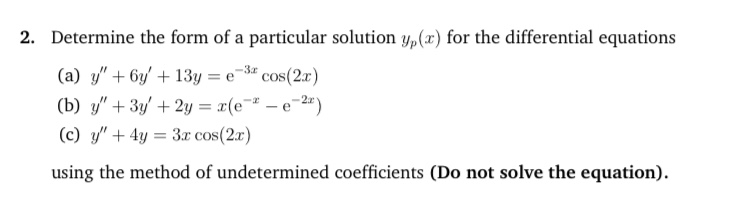# Determine the form of a particular solution y,(x) for the differential equations (a) y" + 6y/ + 13y = e# cos(2a) (b) y" + 3y/ + 2y = r(e - e-2") (c) y" + 4y = 3r cos(2r) -3r using the method of undetermined coefficients (Do not solve the equation).

Questionhelp_outlineImage TranscriptioncloseDetermine the form of a particular solution y,(x) for the differential equations (a) y" + 6y/ + 13y = e# cos(2a) (b) y" + 3y/ + 2y = r(e - e-2") (c) y" + 4y = 3r cos(2r) -3r using the method of undetermined coefficients (Do not solve the equation). fullscreen

### Want to see this answer and more?

Experts are waiting 24/7 to provide step-by-step solutions in as fast as 30 minutes!*

*Response times may vary by subject and question complexity. Median response time is 34 minutes for paid subscribers and may be longer for promotional offers.
Tagged in
Math